# GSEB Class 11 Accounts Important Questions Part 2 Chapter 2 Depreciation Accounts

Gujarat Board GSEB Class 11 Commerce Accounts Important Questions Part 2 Chapter 2 Depreciation Accounts Important Questions and Answers.

## GSEB Class 11 Accounts Important Questions Part 2 Chapter 2 Depreciation Accounts

Answer the following questions in one sentence:

Question 1.
Which type of expense is depreciation?
Depreciation is a non-cash revenue expense.

Question 2.
Which expense is related with depreciation?
Time-related expense is related with depreciation.

Question 3.
What is book value of an asset?
The book value of an asset is the asset’s cost minus the asset’s accumulated depreciation.

Question 4.
Under which method of depreciation, value of asset never become zero?
Under Reducing balance method, value of asset does not become zero at the end of its estimated life.Question 5.
How is the amount of depreciation in Straight-line method?
The amount of depreciation remains identical every year in Straight-line method.

Question 6.
Which method of depreciation is recognised or accepted by all?
Reducing balance method of depreciation is recognised or accepted by all because this method is approved by the income-tax authority.

Question 7.
Which method of depreciation is suitable for assets which have a small and definite useful life?
Straight-line method of depreciation is suitable for assets which have a small and definite useful life.

Question 8.
Which method of depreciation is appropriate for assets which have long term useful life?
Reducing balance method is appropriate for assets which have long term useful life.

Question 9.
Give the formulas to find out annual depreciation (D) under Fixed Instalment method.
Formula no. 1 : D = $$\frac{\mathrm{C}-\mathrm{S}}{\mathrm{N}}$$
Formula no. 2 : D = $$\frac{\mathrm{C} \times \mathrm{R}}{100}$$
Where, D = Annual depreciation
C = Cost price
S = Scrap value
N = Number of years of useful life
R = Rate of depreciation

Question 10.
Give the formula for the rate of depreciation according to Reducing balance method.
Rate of depreciation
= 1 – $$\sqrt[n]{\frac{\text { Scrap value of asset }}{\text { Cost price of asset }}} \times 100$$
Where, n = life in years given for asset.Question 11.
For which assets, straight-line method Of depreciation is appropriate?
Straight-line method of depreciation is appropriate for those assets, whose, useful estimated life can be determined very easily, e.g., Lease-hold properties, copyright, patent, trademark, etc.

Question 12.
For which assets, Reducing balance method of depreciation is appropriate?
Reducing balance method of depreciation is appropriate for those assets which are of long term use and which have balance amount after their use. e.g., Furniture, Fixtures, Plant and Machine, Building, etc.

Question 13.
State the disadvantage of Reducing balance method of depreciation.
Under Reducing balance method, interest on capital employed for asset is not considered.

Question 14.
In which method of depreciation, depreciation is calculated on the opening balance of the asset every year?
In Reducing balance method, depreciation is calculated on the opening balance of the asset every year.

Question 15.
To which account and side, loss on sale of asset is recorded?
Loss on sale of asset is recorded to debit side of Profit and Loss A/c and credit side of asset account.

Question 16.
What is non-depreciable asset?
Asset which has unlimited life or whose estimation of life is not possible, this kind of asset is called a non-depreciable asset, e.g., Land. On non-depreciable asset, depreciation is not calculated.

Question 17.
Which three points should be kept in mind while calculating depreciation according to straight-line method?
For finding out the depreciation according to straight-line method, following three points should be kept in mind :

1. Cost price of the asset,
2. Scrap value of the asset and
3. Number of years of useful life.

Question 18.
Generally, how are the depreciable assets shown in the Balance Sheet?
In the Balance Sheet, the depreciable assets are shown on the assets side at a value obtained by deducting the annual depreciation from the opening balance of the asset.Question 19.
On which assets of the business, depreciation is not to be calculated?
Depreciation is not to be calculated on current and liquid assets of the business, e.g., cash, stock, debtor, etc.

Question 20.
To which account an amount of depreciation is transferred at the end of accounting year?
At the end of every accounting year, the amount of depreciation is transferred to Profit and Loss A/c.

Question 21.
Under which method of depreciation, amount of depreciation change every year?
Under Reducing balance method of depreciation, amount of depreciation changes every year.

Question 22.
Why is depreciation charged?
Depreciation is charged every year to ascertain the true profit or loss; to show the fixed asset at its true value in the Balance Sheet, and to provide for its replacement.

Question 23.
Give the formula of cost price of asset.
For new purchased asset:
Cost price = Purchase price + Transportation cost + Installation cost
For old (Second hand) purchased asset:
Cost price = Purchase price + Expenses incurred to bring the asset in usable forms (repairing expenses).

Question 24.
What is the scrap value of the asset?
The net amount which is expected to be realised on the final disposal of a fixed asset is called scrap value.

Question 25.
Under which method of depreciation, no balance is maintained between depreciation and maintenance expense?
Under straight-line method of depreciation, no balance is maintained between the amount of depreciation and maintenance expense.

Answer the following question in brief:

(1) Harshil Limited purchased a machine on 1-4-2018 for ₹ 1,90,000. Installation expenses amounted to ₹ 15,000 and carriage was ₹ 5,000. The estimated useful life of the machine is 10 years, while its scrap value is estimated to be ₹ 40,000. Calculate the amount of depreciation as per the straight-line method.
Annual depreciation:
D = $$\frac{C-S}{N}$$
Where,
D = Amount of annual depreciation =?
C = Cost price = purchase price + Installation expenses + Carriage charges
= ₹ 1,90,000 + ₹ 15,000 + ₹ 5,000 = ₹ 2,10,000
S = Scrap value = ₹ 40,000
N = Total life in a years = 10 years
D = $$\frac{2,10,000-40,000}{10}=\frac{1,70,000}{10}$$ = ₹ 17,000
∴ The amount of annual deprectatlon is ₹ 17,000.

2. One machine was purchased on 1-4-’11. On 1-4-’17 the opening balance of machine account is 32,000. DepreciatIon on machine is charged at 10 % under straight-line method. Write journal entry for depreciation on 31-3-’18.
First of all. in this sum, cost price of machine will be ascertained.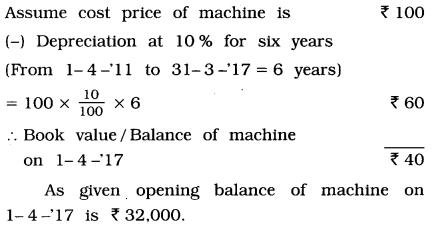If balance of machine is ₹ 40, the cost price is ₹ 100.
∴ If balance of machine is ₹ 32,000, the cost price is (₹)
= $$\frac{32,000 \times 100}{40}$$ = ₹ 80,000
∴ Cost price of machine = ₹ 80,000
Annual Decleration D = $$\frac{\mathrm{C} \times \mathrm{R}}{100}$$
= $$\frac{80,000 \times 10}{100}$$
= ₹ 8,000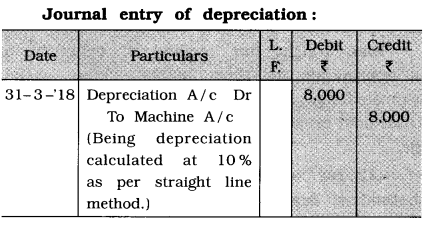3. One machine was purchased on 1-4-’16 for ₹ 50,000. Depreciation on machine is charged at 10 % under reducing balance method. Write journal entry for depreciation on 31-3-’18.
Necessary computation of depreciation amount: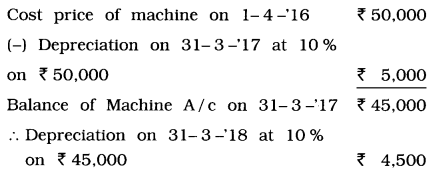Journal entry of depreciation: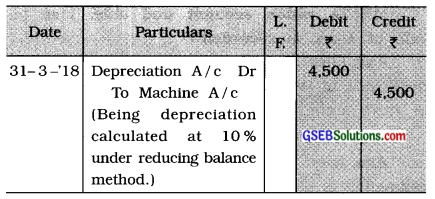4. On 1-4 -‘17 book value of machine was ₹ 86,000. Depreciation is calculated at 20 % under reducing balance method. This machine was sold at 40 % profit on book value on 31- 12 -‘17. From this Information write journal entry for sale of machine.
Ascertain selling price of machine: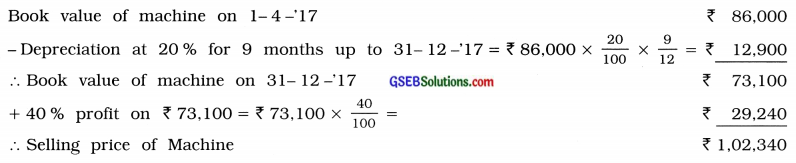Journal entry for sale of machine:

 Date Particulars L. F. Debit ₹ Credit ₹ 31-12-17 Bank A/c Dr 1,02.340 Depreciation A/c Dr 12,900 To Profit and Loss A/c 29,240 To Machinc A/c (Being machine sold at 40% profit.) 86,000

5. Milan Limited charges depreciation on its assets at 8 % under straight-line method. If annual depreciation amount is 12,800, determine cost price of machines.
Cost price of machines:
Annual depreciation D = $$\frac{\mathrm{C} \times \mathrm{R}}{100}$$
∴ Cost price of machines C = $$\frac{\mathrm{D} \times 100}{\mathrm{R}}$$
Where, D = 12,800; R = 8 and C =?
= $$\frac{12,800 \times 100}{8}$$ = 1,60,000
∴Cost price of machines = ₹ 1,60,000

6. Biju Limited has purchased a machine on 1-4-’16. By charging depreciation at 10% under reducing balance method, ₹ 14,400 is recorded as depreciation on 31-3-’18, determine cost price of machine.
Cost price of machine :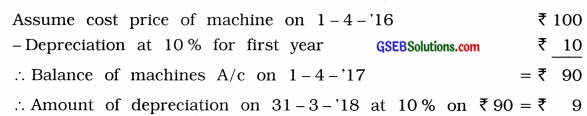Here, amount of depreciation on 31-3-48 is given ₹ 14,400.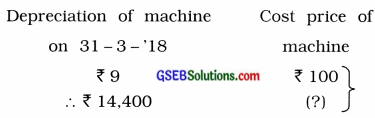=$$\frac{14,400 \times 100}{9}$$ = 1,60,000
∴ Cost price of machine = ₹ 1,60,000

Write the correct option from those given below each question :

1. On which of the following assets of business, depreciation is calculated?
(a) Fixed
(b) Current
(c) Liquid
(d) Land
(a) Fixed

2. On which of the following assets of business, depreciation is not calculated?
(a) Loose tools
(b) Leasehold properties
(c) Stock
(d) Mines
(c) Stock3. Gradual and permanent decrease in the value of an asset is known as …………………….. .
(a) loss
(b) profit
(c) depreciation
(d) scrap value
(c) depreciation

4. Depreciation depends on which of the following factors?
(b) Time
(c) Capital
(d) Debts
(b) Time

5. Under which method, the amount of depreciation change every year?
(a) Straight-line method
(b) Reducing balance method
(c) Revaluation method
(d) Mileage method
(b) Reducing balance method

6. By the amount of depreciation, the value of asset ………………….. .
(a) decreases
(b) increases
(c) becomes zero
(d) never becomes zero
(a) decreases

7. Under which method of depreciation, the value of asset becomes zero?
(a) Reducing balance method
(b) Annuity method
(c) Straight-line method
(d) Depletion unit method
(c) Straight-line method8. Which method of depreciation is very simple and widely used?
(a) Depletion method
(b) Annuity method
(c) Revaluation method
(d) Straight-line method
(d) Straight-line method

9. In which method, every year depreciation is calculated on opening balance of asset at the predetermined rate?
(a) Straight-line method
(b) Depletion method
(c) Written down value method
(d) Mileage method
(c) Written down value method

10. From the viewpoint of accounting, how many methods of recording depreciation are there?
(a) Two
(b) Three
(c) Four
(d) Five
(a) Two

11. Which account is credited to record depreciation under depreciation provision method?
(a) Asset A/c
(b) Depreciation Provision A/c
(c) General Provision A/c
(d) Profit and Loss A/c
(b) Depreciation Provision A/c

12. Out of the following, which asset is a depreciable asset?
(a) Stock
(b) Land
(c) Building
(d) Debtor
(c) Building

13. Under which method of depreciation, interest on capital is ignored?
(a) Compound Interest Method
(b) Fixed Instalment Method
(c) Sinking Fund Method
(d) Depletion Method
(b) Fixed Instalment Method14. What is the other name of Reducing Balance Method?
(a) Written off value method
(b) Written down value method
(c) Written up value method
(d) Written fall value method
(b) Written down value method

15. Out of the following, which asset is not a depreciable asset?
(a) Building
(b) Land
(c) Mines
(d) Gas wells
(b) Land

16. Depreciation is ……………………. related expense.
(a) value
(b) capital
(c) time
(d) income
(c) time

17. Which method of depreciation is used for depreciation for intangible assets?
(a) Straight-line method
(b) Mileage method
(c) Reducing balance method
(d) Revaluation method
(a) Straight-line method

18. Depreciation is one kind of ………………….. .
(a) savings
(b) reserve
(c) provision
(d) liability
(c) provision

19. Under Straight line method, the graph of depreciation of each year remains in …………………………… .
(a) vertical line
(b) horizontal line
(c) falling line
(d) inclined line
(b) horizontal line20. Under which method of depreciation, balance between depreciation amount and repairing expense is maintained?
(a) Annuity method
(b) Revaluation method
(c) Straight-line method
(d) Reducing balance method
(d) Reducing balance method

21. On which balance of asset depreciation is calculated under Reducing balance method?
(a) Closing balance
(b) Opening balance
(c) Average balance
(d) Monthly balance
(b) Opening balance

22. Depreciation is debited to Profit and Loss A/c as ………………………. .
(a) non-cash capital expense
(b) non-cash revenue expense
(c) deferred revenue expense
(b) non-cash revenue expense

23. The amount realised at the end of working life of an asset is known as …………………….. .
(a) residual (scrap) value
(b) market value
(c) written down value
(d) cost value
(a) residual (scrap) value

24. Generally, in the Balance Sheet, the balance of asset is shown after deducting ………………………….. .
(a) scrap value
(b) repairing expenses
(c) depreciation
(d) loss